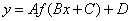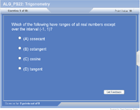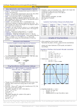HOME COURSES PREVIEW REVIEW ABOUT CONTACTToll-Free Info & Ordering M-F: 9am-5pm (PST): (877) RAPID-1024/7 Technical SupportQUICK TOURMember Login:Rapid Courses Catalog Mathematics in 24 Hours Chemistry in 24 Hours Biology in 24 Hours Physics in 24 HoursMath Survival Weekly
Get the insider's tips and tricks in how to survive your math course and ace the next test. Subscribe the Web's only math weekly newsletter for students and learn:
- How to Study Math Effectively
- How to Take Math Courses Strategically
- How to Solve Math Problems Systematically
- How to Score High in Math Exams
- How to Master Math Rapidly

Enter your name and email below and get started today!
 Your Name * Email *Math Study Lounge These study sheets are for quick review on the subjects. Refer to our rapid courses for comprehensive review.     - Getting Started with Algebra     - Geometry Basics     - How to Solve Math Problems     - Trigonometry Quick Review     - Statistics At-A-Glance     - Calculus PreviewHome » College Algebra

Trigonometry Basics

 Topic Review on "Title": Degree: A degree is a unit of angle measurement equal to 1/360 of a circle’s circumference.  Radian: A radian is a unit of angle measurement that is the ratio of the circle arc cut off by an angle to the central angle.  Sine: Sine on a unit circle is the “y” coordinate for any intersection between a standard angle and the circle.  Cosine: Cosine on a unit circle is the “x” coordinate for any intersection between a standard angle and the circle. Tangent: The ratio of sine to cosine or, in a right triangle, is the ratio of the length of the opposite side to the length of the adjacent side. Reference Angle: A reference angle is the angle between 0 and 90 made with the x axis. Form of a Trigonometric Function:  Trigonometric functions take the formwhere A,B, C, and D are coefficients that change the characteristics of that trigonometric function. Graphs of Trigonometric Functions: Graphs may be affected wildly as follows: Stretching or compressing it along the x-axis Stretching or compressing it along the y-axis Moving it up or down along the y-axis

Rapid Study Kit for "Title":
 Flash Movie Flash Game Flash Card Core Concept Tutorial Problem Solving Drill Review Cheat Sheet"Title" Tutorial Summary : Trigonometry is an important branch of mathematics that uses angles and the definitions of the basic trigonometric functions to solve equations. Angles are given in degrees and conversion to radians is performed using the fact that 180 degrees equals to pi. The basic trigonometric functions and their graphs are shown with the use of examples and and graphical representations. Trigonometric identities are presented and how are they use with reference angles. The sine and cosine functions are defined on a unit circle and a triangle.

 Tutorial Features: Specific Tutorial Features: • The properties of the trigonometric functions are shown in some of the examples. • Measuring angles is presented in the examples. • Step by step explanation of how degrees can be converted to radians and vice versa. Series Features: • Concept map showing inter-connections of new concepts in this tutorial and those previously introduced. • Definition slides introduce terms as they are needed. • Visual representation of concepts • Animated examples—worked out step by step • A concise summary is given at the conclusion of the tutorial.

 "Title" Topic List: `Measuring Angles Definition of angles and radians Comparison of degrees and radians Definition of sine and cosine functions Definition of a tangent function Definition of reciprocal trigonometric functions Trigonometric IdentitiesGraphs of Basic Trigonometric Functions Definition of the form of a trigonometric function`

See all 24 lessons in College Algebra, including concept tutorials, problem drills and cheat sheets: Teach Yourself College Algebra Visually in 24 Hours

 ©2014 Rapid Learning Center. Privacy Policy | Disclaimer Chemistry Survival Publishing, Biology Survival Publishing and Physics Survival Publishing are the divisions of Rapid Learning Inc.